# StructBERT: Incorporating Language Structures into Pre-training for Deep Language Understanding

## StructBERT

### Input Representation and Transformer Encoder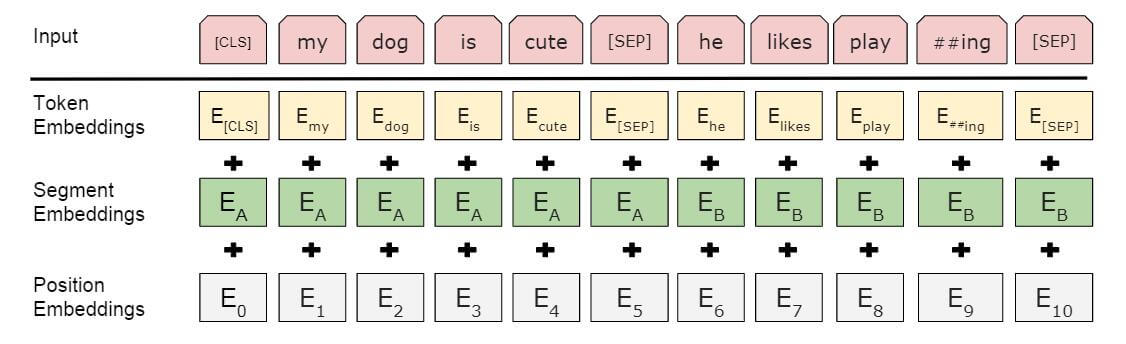$$\mathbf{H}^{l}=\text { Transformer }_{l}\left(\mathbf{H}^{l-1}\right)$$

### Word Structural Objective

$$\arg \max _{\theta} \sum \log P\left(\operatorname{pos}_{1}=t_{1}, \operatorname{pos}_{2}=t_{2}, \ldots, \operatorname{pos}_{K}=t_{K} \mid t_{1}, t_{2}, \ldots, t_{K}, \theta\right)$$
$\theta$ 为参数, $K$ 为打乱顺序的子序列长度.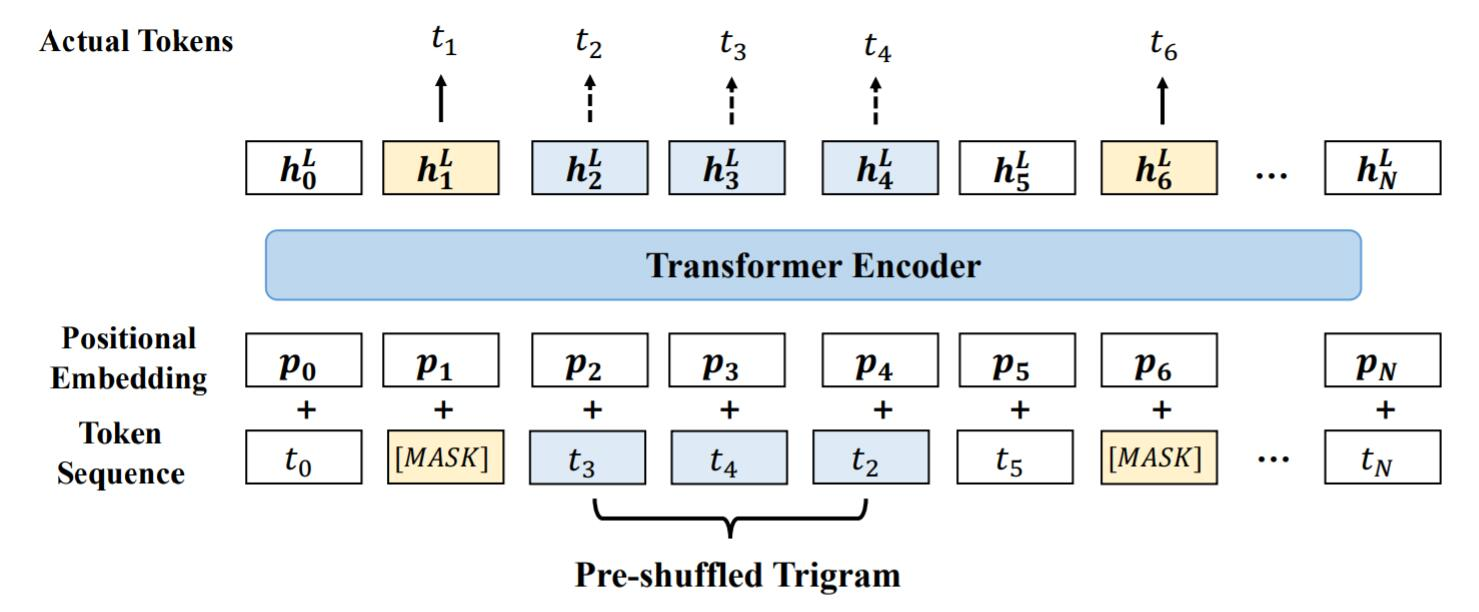Word Structural Objective主要针对单句子任务.

### Sentence Structural Objective

1. $S_2$ 为$S_1$ 的一句, 此时任务为原始的NSP任务.
2. $S_2$ 为$S_1$ 的一句.
3. $S_2$ 为其他文档随机一句.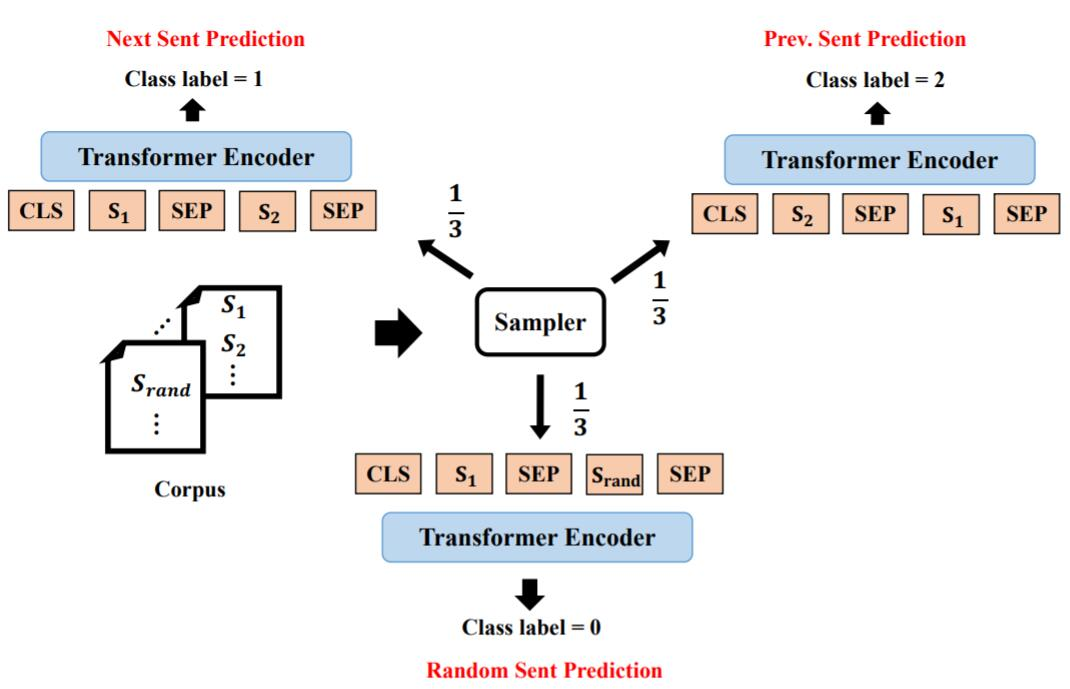Sentence Structural Objective主要针对句子对任务.

## Experiments

### General Language Understanding

#### GLUE benchmark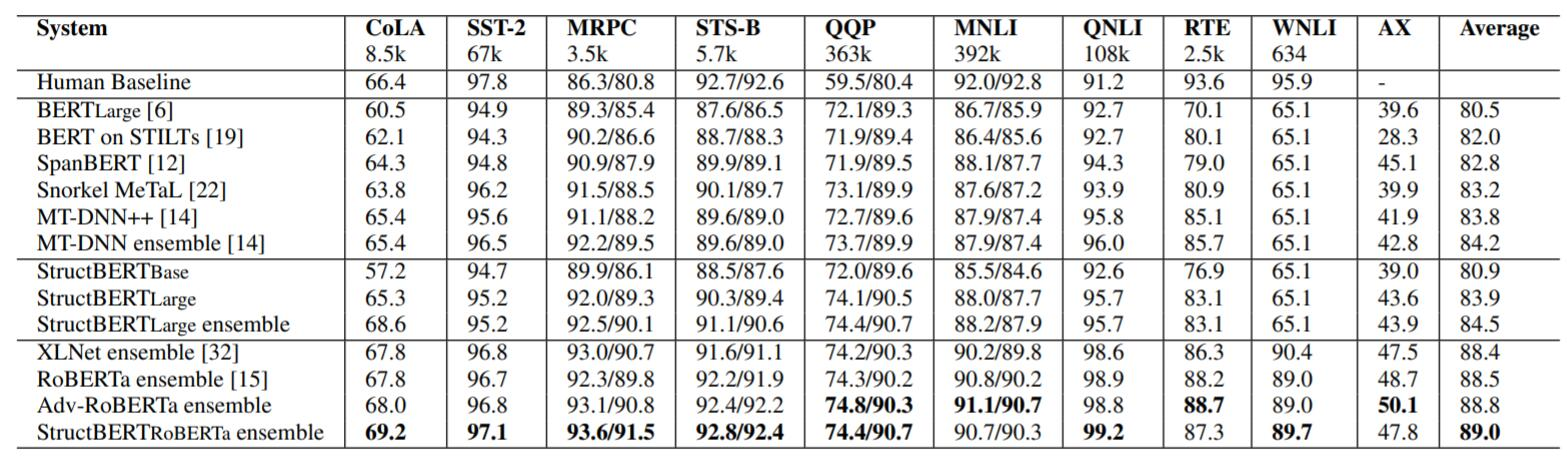StructBERT在GLUE上的平均表现超过了其他的PLM.

#### SNLI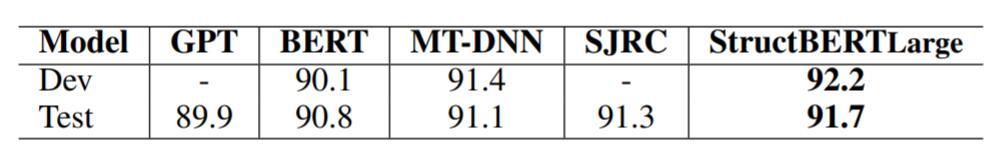### Effect of Different Structural Objectives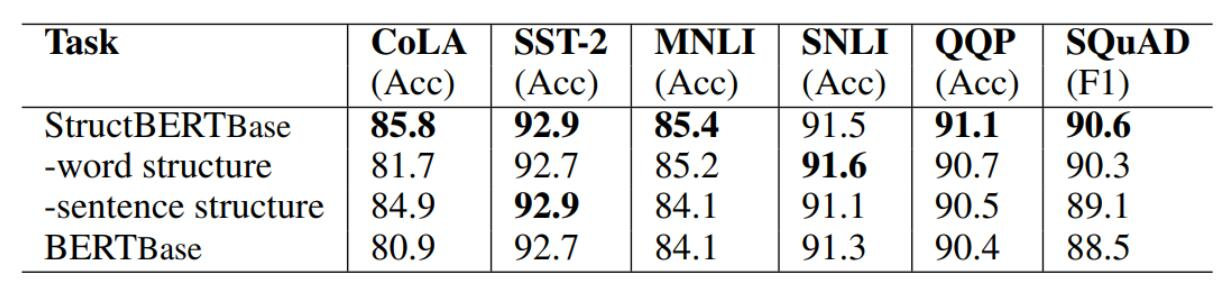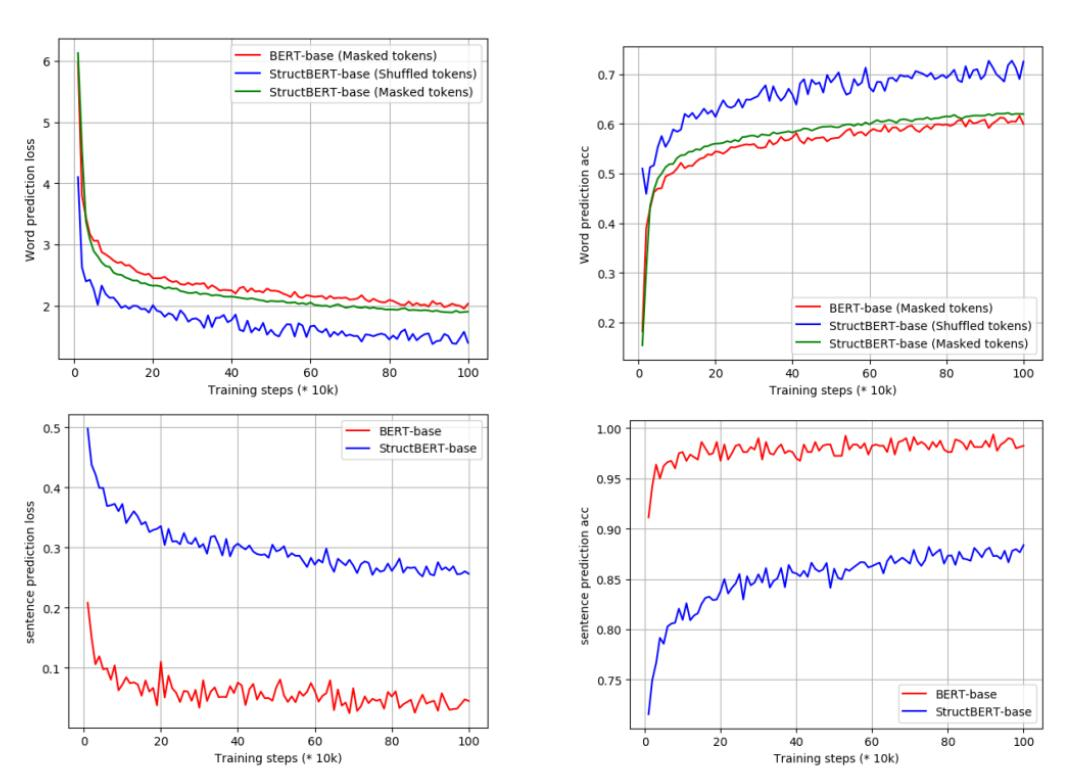## Summary

StructBERT通过在原有任务的基础上从Word Level和Sentence Level添加了两个新的辅助训练任务, 使得BERT能够关注一些语言结构, 想法非常非常简单, 但效果却出乎意料的好.

StructBERT属于对BERT的改进, 没有太多值得进一步的建议.

上一篇SpanBERT: Improving Pre-training by Representing and Predicting Spans

2021-05-13BART和mBART

2021-04-26
目录